# Integral of ln(x)

In this post we will find the antiderivative of ln(x) step-by-step. This is one of those integrals you solve once and never forget. Let’s start!

What is ln(x)?

ln is the natural logarithm, i.e. a logarithm with the number e as base. This means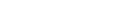Integral of ln(x)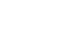Method I (the longest)

Let’s try using the most common integration technique, u-substitution: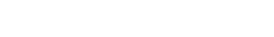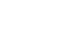Is this good? Not at all! But we can fix things by writing x in terms of u (since we are now integrating with respect to u); how? We let u=ln(x), so x=e^u (property of logarithms: a base x raised to log in base x of an argument y is equal to the argument y).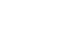We can use integration by parts: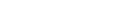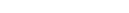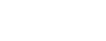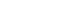Let’s now undo the substitution: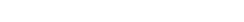Overall: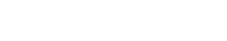You can also factor out the x.

Method II (the shortest)

The best method, however, is integration by parts right away; ln(x) is equal to ln(x)*1. Since we want to find the integral of ln(x), we can’t let dv=ln(x)dx, because this way we would have to integrate it and it would make no sense.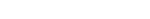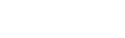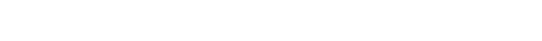And that’s it! Now, try to evaluate this definite integral. If you want, leave the answer in the comments!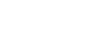If you liked this post give it a like and subscribe for more! It would mean a lot to me!

Join 31 other subscribers.